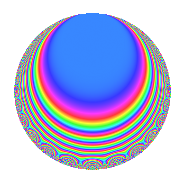# Properties

 Label 4032.2.c.rLevel 4032 Weight 2 Character orbit 4032.c Analytic conductor 32.196 Analytic rank 0 Dimension 8 CM No Inner twists 4

# Related objects

## Newspace parameters

 Level: $$N$$ = $$4032 = 2^{6} \cdot 3^{2} \cdot 7$$ Weight: $$k$$ = $$2$$ Character orbit: $$[\chi]$$ = 4032.c (of order $$2$$ and degree $$1$$)

## Newform invariants

 Self dual: No Analytic conductor: $$32.195682095$$ Analytic rank: $$0$$ Dimension: $$8$$ Coefficient field: 8.0.897122304.10 Coefficient ring: $$\Z[a_1, \ldots, a_{17}]$$ Coefficient ring index: $$2^{12}$$ Sato-Tate group: $\mathrm{SU}(2)[C_{2}]$

## $q$-expansion

Coefficients of the $$q$$-expansion are expressed in terms of a basis $$1,\beta_1,\ldots,\beta_{7}$$ for the coefficient ring described below. We also show the integral $$q$$-expansion of the trace form.

 $$f(q)$$ $$=$$ $$q$$ $$-\beta_{5} q^{5}$$ $$+ q^{7}$$ $$+O(q^{10})$$ $$q$$ $$-\beta_{5} q^{5}$$ $$+ q^{7}$$ $$-\beta_{5} q^{11}$$ $$+ ( -\beta_{4} - \beta_{6} ) q^{13}$$ $$+ \beta_{1} q^{17}$$ $$-\beta_{4} q^{19}$$ $$+ \beta_{2} q^{23}$$ $$+ ( -5 - \beta_{7} ) q^{25}$$ $$-\beta_{3} q^{29}$$ $$-2 q^{31}$$ $$-\beta_{5} q^{35}$$ $$+ ( \beta_{4} + 4 \beta_{6} ) q^{37}$$ $$-\beta_{1} q^{41}$$ $$+ ( -2 \beta_{4} - \beta_{6} ) q^{43}$$ $$+ ( \beta_{1} + \beta_{2} ) q^{47}$$ $$+ q^{49}$$ $$+ ( -10 - \beta_{7} ) q^{55}$$ $$-\beta_{3} q^{59}$$ $$+ ( \beta_{4} + \beta_{6} ) q^{61}$$ $$+ ( \beta_{1} - 3 \beta_{2} ) q^{65}$$ $$+ 5 \beta_{6} q^{67}$$ $$+ ( -2 \beta_{1} + \beta_{2} ) q^{71}$$ $$+ ( 6 - \beta_{7} ) q^{73}$$ $$-\beta_{5} q^{77}$$ $$+ ( -4 + \beta_{7} ) q^{79}$$ $$+ ( \beta_{3} + 2 \beta_{5} ) q^{83}$$ $$+ ( -\beta_{4} + 4 \beta_{6} ) q^{85}$$ $$+ ( -\beta_{1} + 2 \beta_{2} ) q^{89}$$ $$+ ( -\beta_{4} - \beta_{6} ) q^{91}$$ $$-2 \beta_{2} q^{95}$$ $$+ ( 2 - \beta_{7} ) q^{97}$$ $$+O(q^{100})$$ $$\operatorname{Tr}(f)(q)$$ $$=$$ $$8q$$ $$\mathstrut +\mathstrut 8q^{7}$$ $$\mathstrut +\mathstrut O(q^{10})$$ $$8q$$ $$\mathstrut +\mathstrut 8q^{7}$$ $$\mathstrut -\mathstrut 40q^{25}$$ $$\mathstrut -\mathstrut 16q^{31}$$ $$\mathstrut +\mathstrut 8q^{49}$$ $$\mathstrut -\mathstrut 80q^{55}$$ $$\mathstrut +\mathstrut 48q^{73}$$ $$\mathstrut -\mathstrut 32q^{79}$$ $$\mathstrut +\mathstrut 16q^{97}$$ $$\mathstrut +\mathstrut O(q^{100})$$

Basis of coefficient ring in terms of a root $$\nu$$ of $$x^{8}\mathstrut -\mathstrut$$ $$8$$ $$x^{6}\mathstrut +\mathstrut$$ $$51$$ $$x^{4}\mathstrut -\mathstrut$$ $$104$$ $$x^{2}\mathstrut +\mathstrut$$ $$169$$:

 $$\beta_{0}$$ $$=$$ $$1$$ $$\beta_{1}$$ $$=$$ $$($$$$6 \nu^{7} + 17 \nu^{5} + 85 \nu^{3} - 403 \nu$$$$)/663$$ $$\beta_{2}$$ $$=$$ $$($$$$-14 \nu^{7} + 255 \nu^{5} - 1377 \nu^{3} + 4771 \nu$$$$)/663$$ $$\beta_{3}$$ $$=$$ $$($$$$32 \nu^{7} - 204 \nu^{5} + 1632 \nu^{3} - 676 \nu$$$$)/663$$ $$\beta_{4}$$ $$=$$ $$($$$$32 \nu^{6} - 204 \nu^{4} + 1632 \nu^{2} - 2002$$$$)/663$$ $$\beta_{5}$$ $$=$$ $$($$$$40 \nu^{7} - 255 \nu^{5} + 1377 \nu^{3} - 845 \nu$$$$)/663$$ $$\beta_{6}$$ $$=$$ $$($$$$2 \nu^{6} - 16 \nu^{4} + 76 \nu^{2} - 104$$$$)/39$$ $$\beta_{7}$$ $$=$$ $$($$$$4 \nu^{6} + 400$$$$)/51$$
 $$1$$ $$=$$ $$\beta_0$$ $$\nu$$ $$=$$ $$($$$$\beta_{3}\mathstrut +\mathstrut$$ $$\beta_{2}\mathstrut -\mathstrut$$ $$3$$ $$\beta_{1}$$$$)/8$$ $$\nu^{2}$$ $$=$$ $$($$$$-$$$$\beta_{7}\mathstrut -\mathstrut$$ $$6$$ $$\beta_{6}\mathstrut +\mathstrut$$ $$8$$ $$\beta_{4}\mathstrut +\mathstrut$$ $$16$$$$)/8$$ $$\nu^{3}$$ $$=$$ $$($$$$-$$$$4$$ $$\beta_{5}\mathstrut +\mathstrut$$ $$5$$ $$\beta_{3}$$$$)/4$$ $$\nu^{4}$$ $$=$$ $$($$$$4$$ $$\beta_{7}\mathstrut -\mathstrut$$ $$24$$ $$\beta_{6}\mathstrut +\mathstrut$$ $$19$$ $$\beta_{4}\mathstrut -\mathstrut$$ $$38$$$$)/4$$ $$\nu^{5}$$ $$=$$ $$($$$$-$$$$32$$ $$\beta_{5}\mathstrut +\mathstrut$$ $$27$$ $$\beta_{3}\mathstrut +\mathstrut$$ $$5$$ $$\beta_{2}\mathstrut +\mathstrut$$ $$81$$ $$\beta_{1}$$$$)/8$$ $$\nu^{6}$$ $$=$$ $$($$$$51$$ $$\beta_{7}\mathstrut -\mathstrut$$ $$400$$$$)/4$$ $$\nu^{7}$$ $$=$$ $$($$$$204$$ $$\beta_{5}\mathstrut -\mathstrut$$ $$151$$ $$\beta_{3}\mathstrut +\mathstrut$$ $$53$$ $$\beta_{2}\mathstrut +\mathstrut$$ $$453$$ $$\beta_{1}$$$$)/8$$

## Character Values

We give the values of $$\chi$$ on generators for $$\left(\mathbb{Z}/4032\mathbb{Z}\right)^\times$$.

 $$n$$ $$127$$ $$577$$ $$1793$$ $$3781$$ $$\chi(n)$$ $$1$$ $$1$$ $$1$$ $$-1$$

## Embeddings

For each embedding $$\iota_m$$ of the coefficient field, the values $$\iota_m(a_n)$$ are shown below.

For more information on an embedded modular form you can click on its label.

Label $$\iota_m(\nu)$$ $$a_{2}$$ $$a_{3}$$ $$a_{4}$$ $$a_{5}$$ $$a_{6}$$ $$a_{7}$$ $$a_{8}$$ $$a_{9}$$ $$a_{10}$$
2017.1
 1.30421 + 0.752986i −1.30421 + 0.752986i −2.07341 − 1.19709i 2.07341 − 1.19709i 2.07341 + 1.19709i −2.07341 + 1.19709i −1.30421 − 0.752986i 1.30421 − 0.752986i
0 0 0 4.11439i 0 1.00000 0 0 0
2017.2 0 0 0 4.11439i 0 1.00000 0 0 0
2017.3 0 0 0 1.75265i 0 1.00000 0 0 0
2017.4 0 0 0 1.75265i 0 1.00000 0 0 0
2017.5 0 0 0 1.75265i 0 1.00000 0 0 0
2017.6 0 0 0 1.75265i 0 1.00000 0 0 0
2017.7 0 0 0 4.11439i 0 1.00000 0 0 0
2017.8 0 0 0 4.11439i 0 1.00000 0 0 0
 $$n$$: e.g. 2-40 or 990-1000 Embeddings: e.g. 1-3 or 2017.8 Significant digits: Format: Complex embeddings Normalized embeddings Satake parameters Satake angles

## Inner twists

Char. orbit Parity Mult. Self Twist Proved
1.a Even 1 trivial yes
3.b Odd 1 yes
8.b Even 1 yes
24.h Odd 1 yes

## Hecke kernels

This newform can be constructed as the intersection of the kernels of the following linear operators acting on $$S_{2}^{\mathrm{new}}(4032, [\chi])$$:

 $$T_{5}^{4}$$ $$\mathstrut +\mathstrut 20 T_{5}^{2}$$ $$\mathstrut +\mathstrut 52$$ $$T_{11}^{4}$$ $$\mathstrut +\mathstrut 20 T_{11}^{2}$$ $$\mathstrut +\mathstrut 52$$ $$T_{13}^{4}$$ $$\mathstrut +\mathstrut 32 T_{13}^{2}$$ $$\mathstrut +\mathstrut 64$$ $$T_{17}^{4}$$ $$\mathstrut -\mathstrut 44 T_{17}^{2}$$ $$\mathstrut +\mathstrut 52$$ $$T_{23}^{4}$$ $$\mathstrut -\mathstrut 60 T_{23}^{2}$$ $$\mathstrut +\mathstrut 468$$ $$T_{31}$$ $$\mathstrut +\mathstrut 2$$